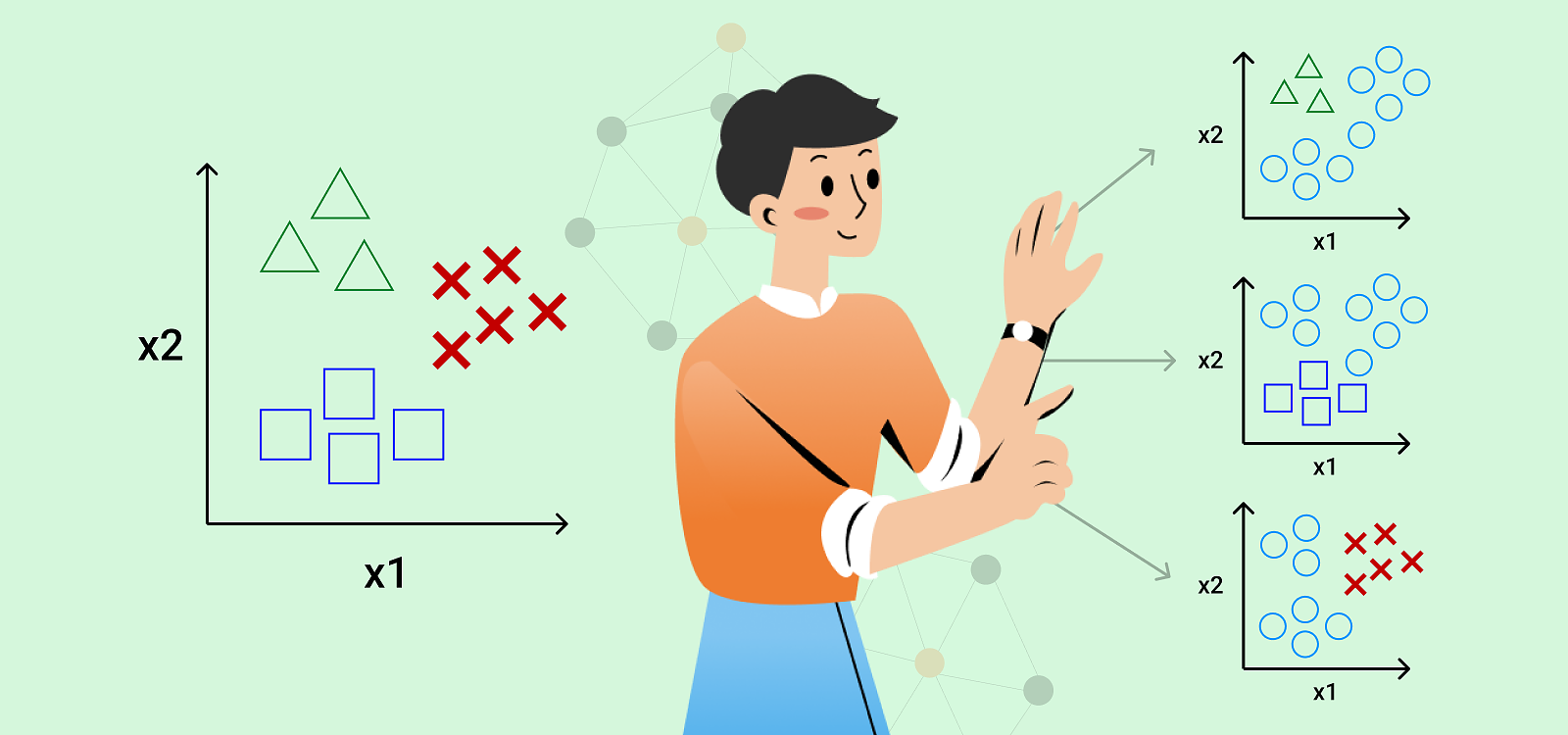August 25, 2022

In machine learning, classification is the problem of assigning a label (also often referred to as a class) to some particular observation. In previous articles, we’ve seen how Smart Blood Analytics Swiss uses the classification for assigning the most probable diagnosis (based on ICD-10 codes) to a given patient based on observed biomarkers of that patient. In this article, we will explore different types of classification problems and the main differences between them.

Probably the simplest form of classification is binary classification, where the task is to assign one of two labels to an observation. The “binary” refers to the fact that the class we are trying to predict has only two possibilities, for example “positive” or “negative” and “yes” or “no”. An example of a binary classification would be to determine whether or not a patient has a certain disease or not.

An extension of binary classification is multiclass classification where the label can have more than two values. When assigning a multiclass label we can either use a classification algorithm that is capable of predicting multiple classes or we can reformulate the problem into multiple binary sub-problems, where each class uses a binary classifier. It is also worth noting that classes can have some underlying structure. The class can be:

• categorical (e.g., A, B, AB, or 0, when predicting the blood type of a patient)
• ordinal (e.g., small, medium or large)
• integer-valued (like heart rate)
• real-valued (like pH level).

The approach used would then depend on this underlying structure. For example, if the classes have a strong ordinal relationship, we might want to reconsider if the problem is really one of classification or if regression might be more suitable.

Due to computational limits, classification is traditionally used to solve only single-label problems, meaning that at most one class is assigned to each observation. However, certain problems require us to assign more than one label to an observation. In addition, each label can be binary or multiclass. Since multiple labels can be assigned to the same observation, we refer to such tasks as multi-label classification. One such task we are trying to solve is predicting diagnoses based on a patient’s blood test results. To solve such a problem, we either need to use specialized versions of standard classification algorithms that support predicting multiple labels or we can transform the problem into a set of multiple binary or multiclass classification problems where for each label a separate binary or multiclass classifier is developed. This approach is often referred to as binary relevance and is probably the most intuitive solution to multi-label tasks. However, the main drawback of binary relevance is that it does not capture any dependence between the labels since it completely ignores possible correlations between them. Another weakness of such an approach arises if the number of labels we can assign to an observation is extremely large, such as predicting a patient’s diagnosis since ICD-10 defines more than 68,000 codes and is continuously being updated.

In this article, we looked at different types of classification tasks and how more complex problems such as multi-label classification can be decomposed into smaller sub-problems and consequently be solved with simpler methods, e.g. binary relevance. We also saw that binary relevance has a couple of drawbacks. In the upcoming articles, we will explore how Smart Blood Analytics Swiss uses more advanced methods to overcome such weaknesses and which help us to boost the predictive performance of our algorithms.

Smart Blood Analytics Swiss SA Höschgasse 25, CH-8008 ZÜRICH, Switzerland